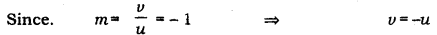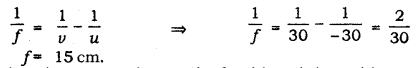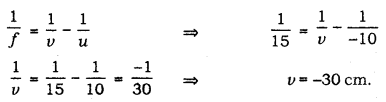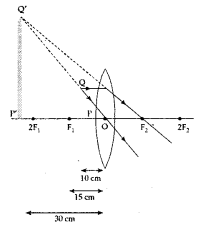# The magnification of an image formed by a lens is -1

The magnification of an image formed by a lens is -1. If the distance between the object and its image is 60 cm, what is the distance of the object from the optical centre of the lens? Find the nature and focal length of the lens. If the object is displaced 20 cm towards the optical centre of the lens, where should the image be formed and what could be its nature? Draw a diagram to justify your answer.

Given, magnification, m= - 1This means that object and image formed is at the same distance from the optical centre and are on the opposite side of the lens. Since it is given that the distance between the object and its image is 60 cm
=> u = - 30 cm and v = 30 cm

Hence, the distance of the object from the optical centre of the lens is 30 cm.
Using lens formula,The given lens is a convex lens as its focal length is positive and the focal length and the lens is 15 cm. If the object is now displaced 20 cm towards the optical centre of the lens that means it is now placed at a distance of 10 cm from the lens this shows that the object is now placed between the optical centre and the focus of the lens. Using lens formulaSo, in this case the image is formed at a distance of 30 cm from the lens on the same side as the object.
The nature of the image formed is(1) virtual
(2) erect
(3) enlarged